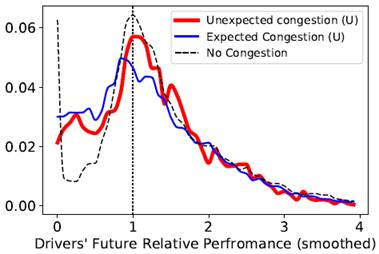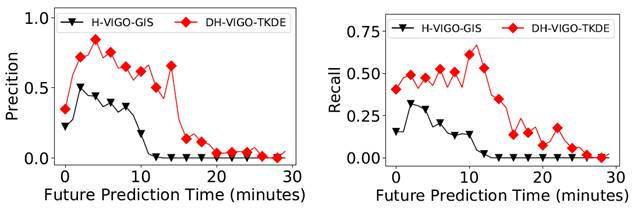Amin Vahedian Khezerlou Research Precise Bayes Classification Algorithm*New   The Bayes Optimal Classifier is shown to have minimal classification error, given the true distribution of the predictors (citation in the article). However, it is almost never the case that we have such a knowledge. Methods such as Naive Bayes and Bayesian Network Classifier aim to estimate the class posteriors by making certain assumptions. Naive Bayes assumes that predictors are distributed with a certain form (usually normal) and are independent of each other. These restrictive assumptions not only reduce accuracy, but also negatively affect explainability. Bayesian Network Classifier does not assume independence. However, it requires that we specify pairwise dependencies beforehand. This task is never straightforward and can quickly become prohibitive with growing dimensionality. In our work we make no assumptions on dependency or distributional form. We define a new formulation of the Bayes Optimal Classifier in discrete space. We propose a novel classification algorithm called Precise Bayes to implement this new formulation. In this method we directly estimate the class posteriors, given the predictors, with almost no parameters to set and no restrictive assumptions. Moreover, the predictions by Precise Bayes are fully explainable. That is, every prediction is an empirical probability calculation of every possible class, given the test point, and the class with the highest probability is chosen as the predicted class.The above figure shows the error rate of Precise Bayes with increasing number of training samples. This is the result of an experiment on synthetic data. Since the distributions are known in this experiment, we can calculate the theoretical minimal error in discrete space. This theoretical minimal error is shown with a dashed line in the figure. The results show that with increasing number of samples, the error rate of Precise Bayes approaches the theoretical limit of classification error. In our recent paper accepted to ICDM 2021 conference, we proposed a lemma proving that Precise Bayes will approach this theoretical limit, and this experiment confirms that proposition. Our argument is that, with more samples our estimated empirical distributions will be closer to true distributions, thus making our error rate be closer to the minimal error rate.   One limitation of Precise Bayes is its reliance on large number of samples. In some cases, Precise Bayes is unable to make predictions for a test point, because the training set did not include a point that is similar enough. Moreover, the memory usage of Precise Bayes grows with increasing number of samples. Even though this growth is sub-linear, it still can create problems. That is because to be more accurate we always need more and more samples, and we cannot have the model grow to unlimited size. Future research will address these issues by making design decisions that curb the memory growth and reduce the need for large number of samples.     Effects of Traffic Congestion on Taxi Driver Work Motivation   In this research, we validate important management theories within a multidisciplinary collaboration to uncover patterns of behavior by drivers after traffic congestion. Specifically, how do taxi drivers perform (in terms of earnings) after experiencing congestion for extended periods of time. We used a massive dataset of taxi GPS records. In this work, our novel Bayesian model made it possible to compare probability distributions of performance, instead of simple linear analysis or comparison of point estimates.The above figure shows probability distributions of taxi drivers' relative performance, following a traffic congestion. Relative performance of 1 means they perform the same as their own average. Numbers higher than 1 mean out-performance and numbers lower than 1 mean under-performance. The analysis of these three distributions show that drivers under-perform themselves after experiencing traffic congestion. Our collaborators used the result of this analysis to examine the validity of work motivation theories. An article on this research will be submitted to the Information Systems Research (ISR) journal.     Predicting Urban Dispersal Events   A dispersal event is the process of an unusually large number of moving objects leaving the same area within a short period of time. Early prediction of dispersal events is important in mitigating congestion and safety risks and making better dispatching decisions for taxi and ride-sharing fleets. Literature of dispersal event prediction solves this problem as a taxi demand prediction problem. It is shown that taxi demand is highly predictable (see article for citation). However, dispersal events are by definition violations of this predictable pattern. Thus, existing methods fail to give accurate predictions of demand in case of dispersal events. There are two main questions in this study: (1) Will there be a dispersal event in the future? If so, (2) what is the demand during the dispersal event?   To answer these questions, we proposed a two-step framework by formulating the Dispersal event prediction problem as a Survival Analysis problem. We call this framework DILSA+. The proposed framework is capable of predicting the occurrence and start time of dispersal events in addition to the abnormal demand in case of such an event. In our formulation, the occurrence of dispersal event is treated as the death event in conventional survival analysis. This formulation uses deep artificial neural networks to estimate the survival function for a target period in the future. Using DILSA+, future dispersal events are predicted with recall of 0.53 and precision of 0.88.The above figure shows an example of a real-world dispersal event predicted by DILSA on March 19, 2016 at Pier 92/94 in Manhattan as a result of a home design exhibition. The second figure shows the survival function during the prediction target period. The vertical line shows the predicted start time of the dispersal anomaly. The third figure shows the predicted pickup counts during the dispersal event. The figure shows that DILSA+ predicts the unexpected high demand, while the baseline DMVST-Net (see article for citation) stays close to the historical average. This work has been published in ACM Transactions on Intelligent Systems and Technology (TIST), after an earlier version was published in the proceedings of the AAAI 2019 conference.     Predicting Urban Gathering Events   We used destination prediction of GPS trajectories to forecast gathering events. Destination prediction is challenging due to complex dependencies among the segments of each trajectory. This challenge has been addressed in the literature by modeling the trajectory as transitions between locations, which are treated as Markovian states (see article for citation). Formulating the trajectory as a Markov process enforces the inherent assumption of independence from the past. In the context of an urban trip, this assumption is severely limiting, because future locations of a traveler strongly depend on its past locations. We relax this limiting assumption and address the resulting computational challenge by proposing a state-of-the-art destination prediction model called Via Location Grouping (VIGO) that efficiently produces destination probabilities for incomplete trajectories. VIGO is a creative implementation of Bayes Optimal Classifier that is designed to specifically work for this problem.Even by using an accurate destination predictor, it is challenging to forecast unexpected gatherings. Because, learning historical patterns of trajectories cannot reliably forecast rare gathering events, as they violate regular patterns by behaving abnormally. To address this challenge, we proposed a Dynamic Hybrid framework, called DH-VIGO, that takes advantage of two VIGO models. This framework is capable of identifying and learning emerging patterns of abnormality in the trajectories, as well as historical patterns. DH-VIGO dynamically decides which pattern (historical vs. recent abnormality) should be used to predict the destination of each incomplete trip. DH-VIGO makes it possible to forecast rare and unexpected gathering events, by pinpointing to the scarce and not-immediately-obvious evidence that exists at current time. The above figure shows the prediction performance of DH-VIGO in forecasting gathering events in future time-steps compared to a baseline we developed specific for this work. This work was published in IEEE Transactions in Data and Knowledge Engineering (TKDE), after an earlier version was published in the proceedings of ACM SIGSPATIAL conference.